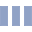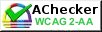## The meaning of Selectivity / Bias / Working range / Sample blank / Within-run precision

Selectivity is an ability in accurately measuring the analyte in the sample, and evaluating the matrix effect on the result value. The study of the selectivity can be done by spiking various amounts of an interference in the sample and the blank, measuring the amount of the analyte, and determining the interference quantity effect on the amount of the analyte. For example, the study of amount of chloride and copper which can interfere the analysis of mercury in the water sample by using Cold Vapour AAS.

Bias can be defined as a difference between an average value of the measurements and a true value. Bias is caused by a systematic error (type B error) from the test method (known as a method bias) and that from the laboratory (known as a laboratory bias). A difference between the average value from inter-laboratory comparison and the true value represents a bias resulting from the test method (or called a method bias). Otherwise, a difference between the average value from the repeatability of the laboratory and the average value from inter-laboratory comparison is called a laboratory bias.

Working range is a range of the analyte concentrations that can be determined by the method showing the accuracy (trueness and precision) within the criteria. Linear range  is a range of the analyte concentrations that can be determined by the method providing the linear relationship between the analyte concentration and the signal intensity. The working range might be either wider or narrower than the linear range; it depends on the ability of the test method  in analyzing the sample.

Sample blank is an analyte-free sample used to study the Method Detection Limit (MDL or Limit of Detection (LOD)) and Limit of Quantitation (LOQ).

Within-run precision is defined as  a closeness of agreement between the test results obtained from successive measurements under the same conditions. For evaluating the within-run precision of a method, it is necessary to assess the test results from the same testing conditions including the same laboratory, the same test sample, the same method, the same staff, the same equipment and the same time period. The within-run precision can be shown as standard deviation (S), relative standard deviation (RSD), or variance, (S2)